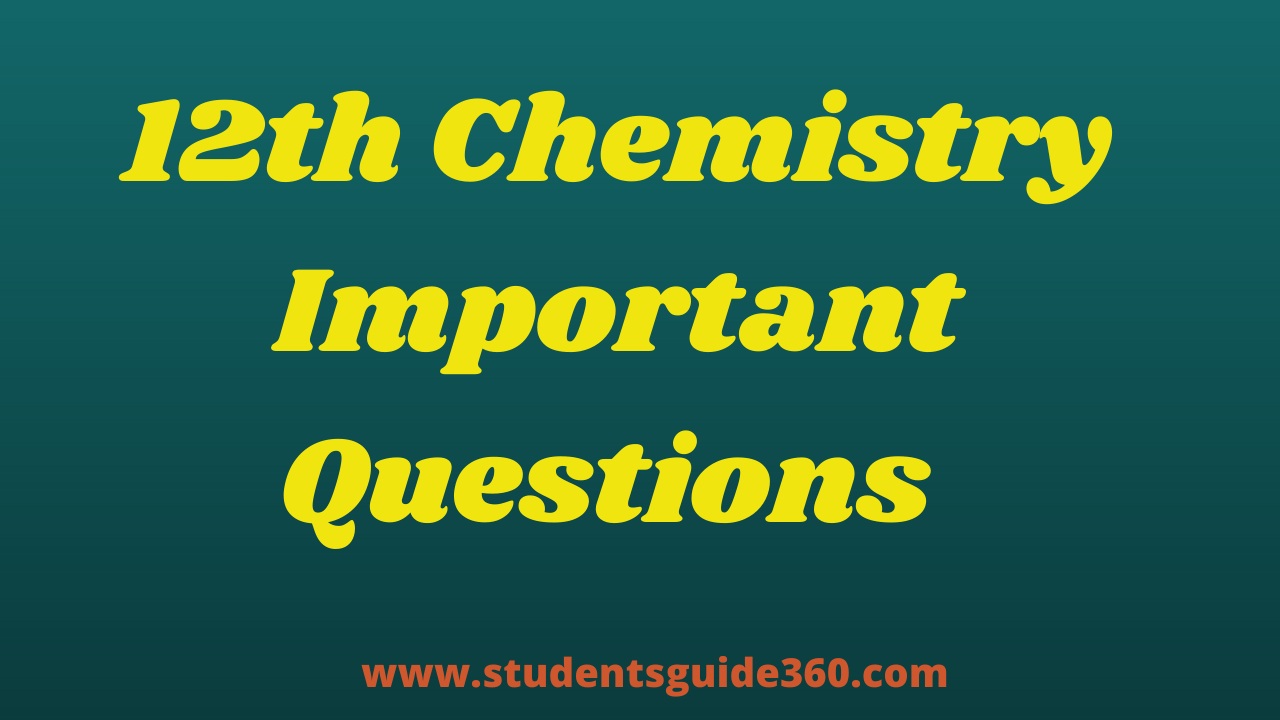# 12th Chemistry Important 1 Marks Solid state

## One Mark Study Material Unit wise as per reduced syllabus (2021-22)

TN 12th Chemistry Important 1 Marks Solid state. 12th Chemistry Important Questions Chapter 6. +2 Chemistry Important 2 Marks,  3 Marks, 5 Marks Questions Based on reduced Syllabus 2021-2022. 12th Free Online Test (MCQs). HSC 12th Chemistry Revision Test Important Questions. 12th Tamil Full Guide.

## Solid state

• 1. An example of metal deficiency defect is Feo
• 2. An ion leaves its regular site and occupies a position in the space between the lattice sites. This defect is called as Frenkel defect
• 3. In a simple cubic cell, each point on a corner is shared by eight unit cells.
• 4. In Bragg’s equation ‘n’ represents order of reflections
• 5. The Bragg’s equation is nꓥ = 2dsin𝜽
• 6. The Co-ordination number of ZnS is 4
• 7. The Co-ordination number of B2O3 is 3
• 8. The Co-ordination number of NaCl is 6
• 9. The Co-ordination number of CsCl is 8
• 10. The crystal structure of Cscl is Body centered cubic
• 11. A regular three dimensional arrangement of identical points in space is called
Unit cell
• 12. An example of frenkel defect is AgBr
• 13. The Metallic solids are good conductors of electricity and heat is produced.
• 14. The solid in which constituents have an orderly arrangement extending over a long range in Crystalline solids.
• 15. The Co-ordination number of each atom in simple cubic, Face centered cubic, Body centered cubic are 6, 2, 8
• 16. Percentage of Schottky defect in VO (Vanadium monoxide) crystal 14%
• 17. Metal excess defect & Metal deficiency defect is non-stoichiometric defect
• 18. Percentage of free space(Vacant) in simple cubic, body centered, Face centered cubic unit cell (Cubic close packing) are 47.69%, 32%,26%
• 19. In AAA type each sphere is arranged in contact with 4 of its neighbors.
• 20.In ABAB… type each sphere is arranged in contact with 6 of its neighbors.
• 21. Three atoms P,Q,R crystallize in a cubic solid lattice having P atoms at corners, Q atom at body center, R atom at face center. The formula of the compound is PQR3
• 22. AB AB AB… is the arrangement of layers in the cubic close packing of spheres.
• 23. The general formula of an ionic compound which crystallizes in body centered cubic structure is AB
• 24. ABC ABC ABC… is the arrangement of layers in a hexagonal close packing of atom.
• 25. If ( rc+ / rA- ) is 0.225 – 0.414, the coordination number is 4
• 26. In which crystal system a ≠ b ≠ c, 𝛼 = 𝛽 = 𝛾 = 900 is Orthorhombic
• 27. In both hcp & ccp arrangements, the coordination number of each sphere is 12
• 28. The co-ordination number of an Octahedral void is 6
• 29. The co-ordination number of an tetrahedral void is 4
• 30. Naphthalene & Anthracene are Non-Polar molecular crystals.
• 31. Glucose is an example for hydrogen bonded molecular crystals
• 32. The crystal with metal excess defect is ZnO
• 33. A unit cell that contains only one lattice point is called Face centered cubic unit cell
• 34. In which crystal system a = b ≠ c, 𝛼 = 𝛽 = 𝛾 = 900 is tetragonal
• 35. Glass is an amorphous solid
• 36. Inner transition elements is an example for atomic solids.
• 37. Diamond is a covalent crystal
• 38. Molecular solids are contain neutral molecules held together by weak vanderwaal’s forces
• 39. Silicon Carbide is an example of Covalent solid
• 40. Solid NH3 & CO2 are example of molecular solids
• 41. Each atom in the corner of the cubic unit cell is shared by how many unit cells? 8
• 42. Feo & ZnO metal show non-stoichiometric defect.
• 43. The co-ordination number of each atom in a simple cubic unit cell is 6
• 44. The no. of atoms belongs to bcc unit cell is 2
• 45. The no. of. Atoms belongs to fcc unit cell is 4
• 46. The atoms the face center is being shared by 2 unit cells.
• 47. The Co-ordination number of Zns is 4
• 48. The Co-ordination number of Cscl is 8
• 49. Metal excess defect possible in KCl
• 50. NaCl, & Diamond are the example of crystalline solid.
• 51. Plastic & Glass are the example of Amorphous solid.
• 52. In naphthalene, constituent molecules are held together by London forces
• 53. Packing efficiency of BCC is 68%
• 54. The radius of Na+ ion is 95 pm and Cl- ion 181 pm. The Co-ordination no. of. Na+ is 6
• 55. The no. of. Close packed sphere is ‘a’ the no. of. Tetrahedral voids generated is equal to 2N
• 56. In FCC unit cell of the edge length is 8√2 pm the radius of the metal atom is
0.04
• 57. The packing efficiency of a fcc structure is 74%
• 58. The arrangement of crystallographic axes and angles respectively in hexagonal crystal system is a = b≠c, 𝜶 = 𝜶 = 𝜷=900 𝜸=1200
• 59. Packing fraction (or) efficiency = [Total volume occupied by spheres in a unit cell/ Volume of the unit cell x 100
• 60. A fcc solid of an element (at.mass 60) has a cube edge of 4A0. Calculate its density 6.2g.cm-3